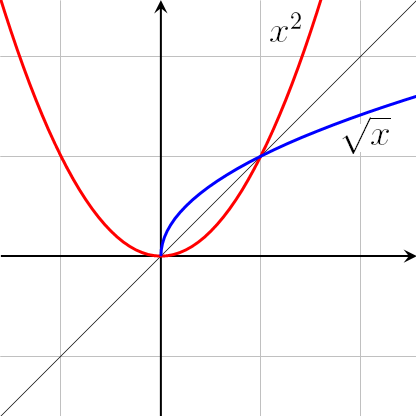# Definition of InverseAn inverse is something that undoes the work of a function or operation.

For example, the inverse of squaring a positive number is taking its square root. The inverse of adding $7$ is subtracting $7$, and the inverse of multiplying by $6$ is dividing by $6$.

### Description

The aim of this dictionary is to provide definitions to common mathematical terms. Students learn a new math skill every week at school, sometimes just before they start a new skill, if they want to look at what a specific term means, this is where this dictionary will become handy and a go-to guide for a student.

### Audience

Year 1 to Year 12 students

### Learning Objectives

Learn common math terms starting with letter I

Author: Subject Coach
You must be logged in as Student to ask a Question.